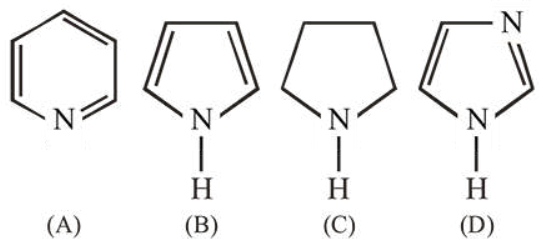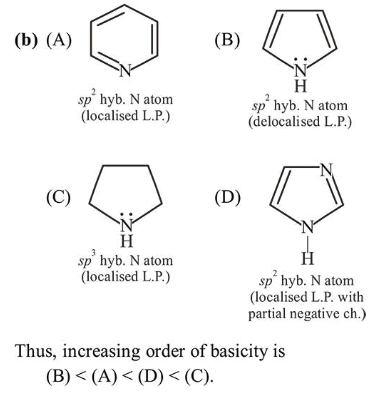# The increasing order of basicity of the following compounds is:

Question:

The increasing order of basicity of the following compounds is:1. $(A)<(B)<(C)<(D)(2)$

2. $(B)<(A)<(D)<(C)$

3. $(\mathrm{D})<(\mathrm{A})<(\mathrm{B})<(\mathrm{C})$

4. $(B)<(A)<(C)<(D)$

Correct Option:

Solution: